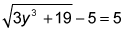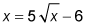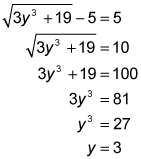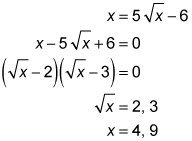##### SAT: 1,001 Practice Questions For DummiesWhen you encounter a question on the SAT Math exam that contains radicals, it won't be as simple as "What's the square root of 9?" Instead, radicals will be just one part of the question.

The following practice questions may look intimidating, but if you know how to solve radicals, you'll breeze through them.

## Practice questions

1. What is the value of y in this equation?A. 2 B. 3 C. 4 D. 5
2. What are the solutions to this equation?A. 2, 3 B. 3, 5 C. 4, 7 D. 4, 9

1. The correct answer is Choice (B). Simplify and solve for y:2. The correct answer is Choice (D). Set the equation equal to 0 and factor it like a quadratic: